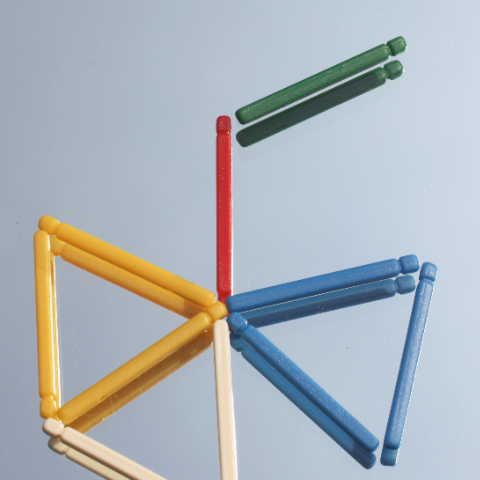# Philosophy of Mathematics (Monday - Week 3, HT19)Gödel's incompleteness theorem has a deep impact on the Logic and the Philosophy of Mathematics.  Somewhat surprisingly it got relatively little attention from the general mathematical public. The feeling was that the "Göodelian statements" are "artificial" and do not come up in the usual mathematical practice. The situation was changed to some extent with the discovery that central problems of Set Theory, like the Continuum Hypothesis, are independent of the usual axioms of Set Theory. It was followed by proofs that many other problems in different areas of Mathematics are independent of the usual axioms.

In spite of that, several authors claimed that these independence results have no effect on the core practice of Mathematics, either because the independent problems are meaningless or that the problems which are considered to be the main stream of the mathematical activity are not independent .I shall argue that these positions are problematic.

I shall also consider whether the phenomena of independence in Mathematics could have any relevance to the use of mathematical models in Science.  While we do not have concrete examples of a scientific theory, (say in theoretical physics) that is sensitive to the truth of a mathematical statement which is independent, I shall argue that it is not impossible. The issue raises some basic questions about the application of Mathematics in Science.

Philosophy of Mathematics Seminar Convenors: Daniel Isaacson and Volker Halbach